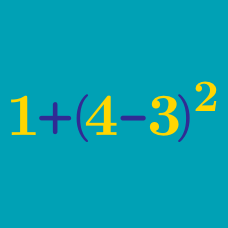Everyday Math

# Exponents

Solve for $N$:

$3 ^ N = 729.$

What is the cube root of 512?

What is the value of $(10 + 2 ) ^ 2$?

What is the value of $10^2$?

What is the value of $3^4$?

×